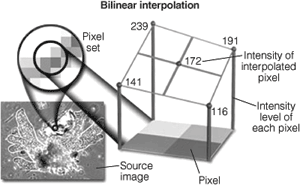# Understanding image-interpolation techniques

In many image-processing applications, digital images must be zoomed to enlarge image details and highlight any small structures present.In many image-processing applications, digital images must be zoomed to enlarge image details and highlight any small structures present. This is done by making multiple copies of the pixels in a selected region of interest (ROI) within the image. Several algorithms are used to perform such an operation. The simplest and most accurate, known as a discrete replicating zoom, displays multiple copies of each pixel in the ROI. Because this algorithm operates in discrete steps, it can produce zoomed images at integral zoom factors of 2X, 4X, and higher. Depending on the spatial resolution of the image, individual pixels can become apparent at 4X or higher zoom factors.

Fractionally zoomed images can also be obtained by varying the number of copies made of each pixel in the ROI. The most common algorithm is the fractional replicating zoom, which operates by copying pixels from the source image into the zoomed image based upon an inexact spatial correspondence between the two. In effect, pixel addresses in the source image are calculated fractionally, based on the ratio between the zoomed image dimensions and the source image dimensions. Because the calculated pixel address is fractional, the effect of this fractional part can be determined by a number of interpolation methods.

#### Interpolation methods

One of the simplest interpolation algorithms is nearest-neighbor interpolation. In this method, the fractional part of the pixel address is discarded, and the pixel brightness value at the resulting integral address in the source image is copied to the zoomed image. Because of the inexactness of the spatial correspondence between the two images, more copies will be made of certain pixels in the source image than of others. This can result in spatial distortion of features in the zoomed image, and nearest-neighbor interpolation is therefore unreliable for measurement purposes. For integral zoom factors that are even (such as 2X and 4X), nearest-neighbor interpolation produces the same results as the discrete replicating zoom algorithm.

An interpolation technique that reduces the visual distortion caused by the fractional zoom calculation is the bilinear interpolation algorithm, where the fractional part of the pixel address is used to compute a weighted average of pixel brightness values over a small neighborhood of pixels in the source image. Bilinear interpolation produces pseudoresolution that gives a more aesthetically pleasing result, although this result is again not appropriate for measurement purposes (see Fig. 1).Figure 1. To reduce the visual distortion caused by fractional zoom, bilinear interpolation uses the fractional part of the pixel address to compute a weighted average of pixel brightness values over a small neighborhood of pixels. This produces a more esthetically pleasing result, although this result is not appropriate for measurement purposes.Click here to enlarge image

The technique of bicubic interpolation produces less blurring of edges and other distortion artifacts than bilinear interpolation, but is more computationally demanding (see Fig. 2). Bicubic interpolation involves fitting a series of cubic polynomials to the brightness values contained in a 4 × 4 array of pixels surrounding the calculated address. First, four cubic polynomials, F(i) (where i = 0, 1, 2, 3), are fitted to the control points in the y-direction (the choice of starting direction is arbitrary). Next, the fractional part of the calculated pixel’s address in the y-direction is used to fit another cubic polynomial in the x-direction, based on the interpolated brightness values that lie on the curves. Substituting the fractional part of the calculated pixel’s address in the x-direction into the resulting cubic polynomial then yields the interpolated pixel’s brightness value. Such bicubic interpolation has found use in many commercial software packages such as Adobe Photoshop.Figure 2. Bicubic interpolation produces less blurring of edges and other distortion artifacts than bilinear interpolation but is more computationally demanding. The technique involves fitting a series of cubic polynomials to the brightness values contained in a 4 × 4 array of pixels surrounding the calculated address.Click here to enlarge image

The choice of polynomial used in the bicubic interpolation algorithm can have a significant impact on the accuracy and visual quality of the interpolated image. Splines are piecewise polynomial functions that are often used in bicubic interpolation algorithms. A significant requirement of the splines used for bicubic interpolation is that they should always interpolate the brightness values of the pixels contained in the 4 × 4 control grid. Because many of the spline functions have control parameters that are subject to the choice of the programmer, reproducibility becomes an issue when comparing the results of bicubic interpolation algorithms between images.

Fractal interpolation, which is based upon self-similar or fractal functions, is popular with some programmers because the technique prevents excessive smoothing of image details and appears to provide additional detail at large zoom factors. Others have developed hybrid approaches by combining wavelets with fractals. However, the additional detail is not real, and so fractal interpolation is not appropriate for measurement.

When accuracy is the goal, more precise algorithms, such as the kriging technique, should be used. Originally developed by French mathematician Georges Matheron based on the Master’s thesis of Daniel Gerhardus Krige, kriging encompasses a family of interpolation algorithms based on a generalized least-squares algorithm that uses plots of spatial statistics or variograms as weighting functions. An advantage of kriging is that the estimated values have a minimum and quantifiable error associated with them.Click here to enlarge image

null

More in Boards & Software